# SSC CHSL Online Practice Test Free

### Quantitative Aptitude (Mathematics)

Q.1: A sum of ₹ x is divided among A, B and C such that the ratio of the shares of A and C is 8 : 7 and that of B and C is 3 : 2. If the difference between the shares of A and B is ₹ 240, then what is the value of x ?
(A) 2544
(B) 2580
(C) 2490
(D) 2448

Ans : (D) 2448

Q.2: If a pair of shoes marked at ₹350 is offered at ₹308, then what will be the discount percentage?
(A) 42%
(B) 13.6%
(C) 12%
(D) 14%

Ans : (C) 12%

Q.3: In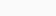$\triangle ABC,$ AD is the bisector of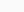$\angle A$ meeting BC at D, If AB = 15 cm, BC = 10 cm and the length of BD is 2 cm less than that of DC, then the length of AC is:
(A) 18.5 cm
(B) 18 cm
(C) 16 cm
(D) 22.5 cm

Ans : (D) 22.5 cm

Q.4: Simplify the following expression.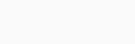$\dfrac{13\frac 79\div\frac23 \text{of} \frac13 + \frac23\times 3}{\frac 75 \text{of} 325 - 20 + 7}$
(A)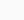$\frac{40}{1989}$
(B)$\frac{31}{2210}$
(C)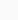$\frac{32}{221}$
(D)$\frac{33}{217}$

Ans : (C)$\frac{32}{221}$

Q.5: The value of cosec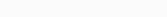$(58^o + \theta)-sec(32^o - \theta)$ + sin15osin35osec55osin30osec75o is :
(A) 1
(B) 2
(C)$\frac12$
(D) 0

Ans : (C)$\frac12$

Q.6: Some persons went on an outstation tour. The histogram shows their ages. How many persons are less than 20 years of age?

(A) 22
(B) 31
(C) 25
(D) 18

Ans : (A) 22

Q.7: Study the given and answer the question that follows.

The total production of paper by company B in 2015 and 2017 to 2019 was what percentage less than 90% of the total production of paper by company A in 2014 to 2019 (correct to one decimal place)?
(A) 22.4%
(B) 19.6%
(C) 21.2%
(D) 17.5%

Ans : (D) 17.5%

Q.8: In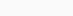$\triangle ABC, D$ and E are points on AB and BC, respectively, such that DE is parallel to AC. If DE = 3 cm. AC = 5 cm and the area of trapezium ACED = 32 cm2 , then what will be the area of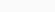$\triangle BDE$ ?
(A)$\frac {144}{17}$cm2
(B)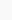$\frac {48}{5}$cm2
(C) 16 cm2
(D) 18 cm2

Ans : (D) 18 cm2

Q.9: Study the given pie chart and answer the question that follows.
Total number of students admitted in a college = 700.
Distribution of the percentage of students in various caurses.

Percentage-wise distribution of the number of boys.

(A) 3 : 7
(B) 4 : 7
(C) 3 : 5
(D) 2 : 5

Ans : (C) 3 : 5

Q10: In a circle with centre O and radius 6.5 cm, a chord AB is at a distance 2.5 cm from the centre. If tangents at A and B intersect at P, then find the distance of P from the centre.
(A) 16.9 cm
(B) 18 cm
(C) 15 cm
(D) 17 cm

Ans : (A) 16.9 cm

Q.11: What is the compound interest (in ₹) on a sum of ₹46,000 for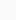$2\frac 25$ years at 15% per annum, interest being compounded annually ( nearest to a ₹) ?
(A) 18,458
(B) 18,485
(C) 19,485
(D) 19,458

Ans : (B) 18,485

Q.12: If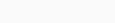$\sqrt x + \frac {1}{\sqrt x} =2\sqrt{3}$, then what will be the value of x4 +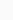$\frac {1}{x^4}$?
(A) 10402
(B) 9606
(C) 9602
(D) 10406

Ans : (C) 9602

Q.13: If 3x – 2y + 3 = 0 , then what will be the value of 27 x3 + 54xy + 30 – 8y3 ?
(A) -27
(B) 57
(C) -57
(D) 3

Ans : (D) 3

Q.14: Chords AB and CD of a circle meet at point P (outside the circle), when produced, If AB = 9 cm, PB =$\frac 13$ AB and CD = 5 cm, then the length of PD (in cm ) is:
(A) 7
(B) 6
(C) 5
(D) 4

Ans : (D) 4

Q.15: By selling an article for ₹3,150, a shopkeeper earns 5% profit. For how much money should he sell the article in order to gain 8% ?
(A) ₹3,240
(B) ₹3,180
(C) ₹3,200
(D) ₹3,250

Ans : (A) ₹3,240

Q.16: Given that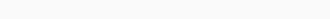$3\sqrt{3x^3} - 8y^3 = (\sqrt3x + Ay) (3x^2 + By^2 + Cxy)$ , the value of (A2 + B2 – C2) is:
(A) 4
(B) 8
(C) 12
(D) 0

Ans : (B) 8

Q.17: A solid metallic sphere of radius 12 cm is melted and recast in the form of small spheres of radius 2 cm. How many small spheres are formed ?
(A) 24
(B) 96
(C) 864
(D) 216

Ans : (D) 216

Q.18: A tap can fill a tank in 4 hours. Another tap can fill the same tank in 6 hours. If both the taps are opened at the same time, then in how much time will the empty tank be filled completely?
(A) 2 h 30 min
(B) 2 h 24 min
(C) 3 h
(D) 2 h

Ans : (B) 2 h 24 min

Q.19: If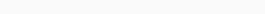$tan 3 \theta = sin45^o.cos45^o + cos60^o \text{and} 3\theta$ is an acute angle, then what will be the value of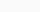$sin4 \theta$?
(A)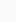$\frac {\sqrt 3} {2}$
(B)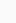$\frac {1} {\sqrt2}$
(C)$\frac 12$
(D) 1

Ans : (A)$\frac {\sqrt 3} {2}$

Q.20: A boat can go 15 km downstream and 8 km upstream in 2 h. It can go 20 km downstream and 12 km upstream in 2 h 50 min. What is the speed (in km/h ) of the boat while going downstream?
(A) 16
(B) 15
(C) 20
(D) 18

Ans : (B) 15

Q.21: Study the given pie chart and answer the question that follows.
The pie chart shows the distribution (degree wise) of the number of computers sold by a shopkeeper during five months.

If the difference between the numbers of computers sold in May and the numbers of computers sold in February is x, then the value of x will be:
(A) 450
(B) 480
(C) 540
(D) 420

Ans : (B) 480

Q.22: The average of 40 numbers is 48.2. The average of the first 15 numbers is 45 and that of the next 22 numbers is 50.5. The 38th and 40th numbers ?
(A) 48
(B) 49
(C) 47.5
(D) 48.5

Ans : (C) 47.5

Q.23: If the five-digit number 457 ab is divisible by 3, 7 and 11, then what is the value of a2 + b2 – ab ?
(A) 49
(B) 36
(C) 24
(D) 33

Ans : (A) 49

Q.24:
(A) 62.5%
(B) 37.98%
(C) 61.25%
(D) 51.6%

Ans : (C) 61.25%

Q.25: If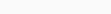$tan \theta + cot \theta = 3$ , then what will be the value of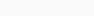$tan^2 \theta + cot^2 \theta$?
(A) 1
(B) 7
(C) 11
(D) -1